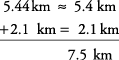## Precision

The word precision refers to the degree of exactness of a measurement, that is, how fine the measurement is. The smaller the unit of measure used, the more precise is the measurement. Keep in mind that the precision of a measurement has nothing to do with the size of the numbers, only with the unit used.
##### Example 1

Which of the following measurements is more precise?

##### 5.4 mm or 3.22 mm 1 m or 1 km 3 ft or 11 in 3.67 m or 5.1 m 5.69 cm or 9.99 cm

1. The smallest unit in 5.4 mm is 0.4, and the smallest unit in 3.22 mm is 0.02, so 3.22 mm is more precise.

2. Meter (m) is a smaller unit than kilometer (km), so 1 m is more precise.

3. Inch (in) is a smaller unit than foot (ft), so 11 inches is more precise.

4. 3.67 goes to the hundredths, and 5.1 goes to the tenths, so 3.67 m is more precise.

##### 5. Each one goes to the hundredths place, so they have the same precision

When adding or subtracting two measures, you cannot be more precise than the least precise unit being used. In other words, the unit being used in the answer should be the same as the less precise of the units used in the two measurements.

##### Example 2

Perform the indicated operation and give the answer in the less precise unit.

1. 5.44 km + 2.1 km

2. 32.77 g – 12 g

First round off the more precise number and then calculate.

1.2.